Tamil Nadu Board of Secondary EducationHSC Science Class 12

# Tamil Nadu Board Samacheer Kalvi solutions for Class 12th Physics Volume 1 and 2 Answers Guide chapter 1 - Electrostatics [Latest edition]

#### Chapters## Solutions for Chapter 1: Electrostatics

Below listed, you can find solutions for Chapter 1 of Tamil Nadu Board of Secondary Education Tamil Nadu Board Samacheer Kalvi for Class 12th Physics Volume 1 and 2 Answers Guide.

Evaluation
Evaluation [Pages 71 - 78]

### Tamil Nadu Board Samacheer Kalvi solutions for Class 12th Physics Volume 1 and 2 Answers Guide Chapter 1 Electrostatics Evaluation [Pages 71 - 78]

#### Multiple choice questions

Evaluation | Q I. 1. | Page 71

Two identical point charges of magnitude –q are fixed as shown in the figure below. A third charge +q is placed midway between the two charges at the point P. Suppose this charge +q is displaced a small distance from the point P in the directions indicated by the arrows, in which direction(s) will +q be stable with respect to the displacement?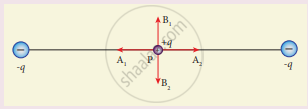• A1 and A2

• B1 and B2

• both directions

• No stable

Evaluation | Q I. 2. | Page 71

Which charge configuration produces a uniform electric field?

• point charge

• uniformly charged infinite line

• uniformly charged infinite plane

• uniformly charged spherical shell

Evaluation | Q I. 3. | Page 71

What is the ratio of the charges |"q"_1/"q"_2| for the following electric field line pattern?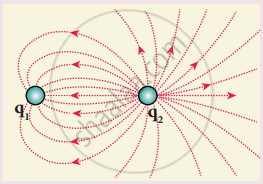• $\frac{1}{5}$

• $\frac{25}{11}$

• 5

• $\frac{11}{25}$

Evaluation | Q I. 4. | Page 71

An electric dipole is placed at an alignment angle of 30° with an electric field of 2 × 105 NC-1. It experiences a torque equal to 8 Nm. The charge on the dipole if the dipole length is lcm is ______.

• 4 mC

• 8 mC

• 5 mC

• 7 mC

Evaluation | Q I. 5. | Page 71

Four Gaussian surfaces are given below with charges inside each Gaussian surface. Rank the electric flux through each Gaussian surface in increasing order.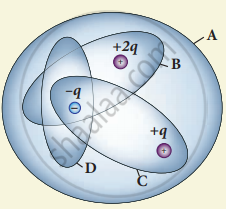• D < C < B < A

• A < B = C < D

• C < A = B < D

• D > C > B > A

Evaluation | Q I. 6. | Page 72

The total electric flux for the following closed surface which is kept inside water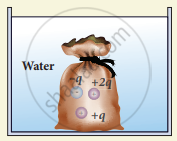• (80"q")/ε_0

• ("q")/(40ε_0)

• ("q")/(80ε_0)

• ("q")/(160ε_0)

Evaluation | Q I. 7. | Page 72

Two identical conducting balls having positive charges q1 and q2 are separated by a center to center distance r. If they are made to touch each other and then separated to the same distance, the force between them will be ______.

• less than before

• same as before

• more than before

• zero

Evaluation | Q I. 8. | Page 72

Rank the electrostatic potential energies for the given system of charges in increasing order.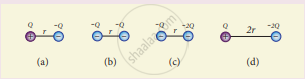• 1 = 4 < 2 < 3

• 2 = 4 < 3 < 1

• 2 = 3 < 1 < 4

• 3 < 1 < 2 < 4

Evaluation | Q I. 9. | Page 72

An electric field vec"E" = 10 xx hat"i" exists in a certain region of space. Then the potential difference V = Vo – VA, where Vo is the potential at the origin and VA is the potential at x = 2 m is:

• 10 V

• - 20 V

• + 20 V

• - 10 V

Evaluation | Q I. 10. | Page 72

A thin conducting spherical shell of radius R has a charge Q which is uniformly distributed on its surface. The correct plot for electrostatic potential due to this spherical shell is

•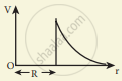•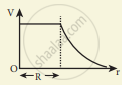•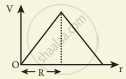•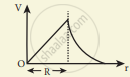Evaluation | Q I. 11. | Page 72

Two points A and B are maintained at a potential of 7 V and -4 V respectively. The work done in moving 50 electrons from A to B is ______.

• 8.80 × 10-17 J

• - 8.80 × 10-17 J

• 4.40 × 10-17 J

• 5.80 × 10-17 J

Evaluation | Q I. 12. | Page 72

If the voltage applied on a capacitor is increased from V to 2V, choose the correct conclusion.

• Q remains the same, C is doubled

• Q is doubled, C doubled

• C remains same, Q doubled

• Both Q and C remain same

Evaluation | Q I. 13. | Page 73

A parallel plate capacitor stores a charge Q at a voltage V. Suppose the area of the parallel plate capacitor and the distance between the plates are each doubled then which is the quantity that will change?

• Capacitance

• Charge

• Voltage

• Energy density

Evaluation | Q I. 14. | Page 73

Three capacitors are connected in a triangle as shown in the figure. The equivalent capacitance between points A and C is ______.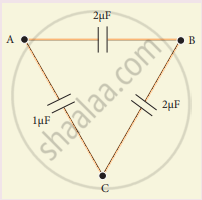• 1 μF

• 2 μF

• 3 μF

• 1/4 μF

Evaluation | Q I. 15. | Page 73

Two metallic spheres of radii 1 cm and 3 cm are given charges of -1 × 10-2 C and 5 × 10-2 C respectively. If these are connected by a conducting wire, the final charge on the bigger sphere is ______.

• 3 × 10-2 C

• 4 × 10-2 C

• 1 × 10-2 C

• 2 × 10-2 C

Evaluation | Q II. 1. | Page 73

What is meant by quantisation of charges?

Evaluation | Q II. 2. | Page 73

Write down Coulomb’s law in vector form and mention what each term represents.

Evaluation | Q II. 3. | Page 73

What are the differences between the Coulomb force and the gravitational force?

Evaluation | Q II. 4. | Page 73

Write a short note on superposition principle.

Evaluation | Q II. 5. | Page 73

Define ‘electric field’.

Evaluation | Q II. 6. | Page 73

What is mean by ‘electric field lines’?

Evaluation | Q II. 7. | Page 73

The electric field lines never intersect. Justify

Evaluation | Q II. 8. | Page 73

Define ‘electric dipole’.

Evaluation | Q II. 8. | Page 73

Give the expression for the magnitude of its electric dipole moment and the direction.

Evaluation | Q II. 9. | Page 73

Write the general definition of electric dipole moment for a collection of point charge.

Evaluation | Q II. 10. | Page 73

Define electrostatic potential.

Evaluation | Q II. 11. | Page 73

What is an equipotential surface?

Evaluation | Q II. 12. | Page 73

What are the properties of an equipotential surface?

Evaluation | Q II. 13. | Page 73

Give the relation between electric field and electric potential.

Evaluation | Q II. 14. | Page 73

Define ‘electrostatic potential energy’.

Evaluation | Q II. 15. | Page 73

Define ‘electric flux’.

Evaluation | Q II. 16. | Page 73

What is meant by electrostatic energy density?

Evaluation | Q II. 17. | Page 74

Write a short note on ‘electrostatic shielding’.

Evaluation | Q II. 18. | Page 74

What is polarisation?

Evaluation | Q II. 19. | Page 74

What is dielectric strength?

Evaluation | Q II. 20. | Page 74

Define ‘capacitance’. Give its unit.

Evaluation | Q II. 21. | Page 74

What is corona discharge?

Evaluation | Q III. 1. | Page 74

Discuss the basic properties of electric charges.

Evaluation | Q III. 2. | Page 74

Explain in detail Coulomb’s law and its various aspects.

Evaluation | Q III. 3. | Page 74

Define ‘electric field’.

Evaluation | Q III. 3. | Page 74

Discuss various aspects of the electric field.

Evaluation | Q III. 4. | Page 74

Calculate the electric field due to a dipole on its axial line and equatorial plane.

Evaluation | Q III. 5. | Page 74

Derive an expression for the torque experienced by a dipole due to a uniform electric field.

Evaluation | Q III. 6. | Page 74

Derive an expression for electrostatic potential due to a point charge.

Evaluation | Q III. 7. | Page 74

Derive an expression for electrostatic potential due to an electric dipole.

Evaluation | Q III. 8. | Page 74

Obtain an expression for potential energy due to a collection of three point charges which are separated by finite distances.

Evaluation | Q III. 9. | Page 74

Derive an expression for electrostatic potential energy of the dipole in a uniform electric field.

Evaluation | Q III. 10. | Page 74

Obtain Gauss law from Coulomb’s law.

Evaluation | Q III. 11. | Page 74

Obtain the expression for electric field due to an infinitely long charged wire.

Evaluation | Q III. 12. | Page 74

Obtain the expression for electric field due to an charged infinite plane sheet.

Evaluation | Q III. 13. | Page 74

Obtain the expression for electric field due to an uniformly charged spherical shell.

Evaluation | Q III. 14. | Page 74

Discuss the various properties of conductors in electrostatic equilibrium.

Evaluation | Q III. 15. | Page 74

Explain the process of electrostatic induction.

Evaluation | Q III. 16. | Page 74

Explain dielectrics in detail and how an electric field is induced inside a dielectric.

Evaluation | Q III. 17. | Page 74

Obtain the expression for capacitance for a parallel plate capacitor.

Evaluation | Q III. 18. | Page 74

Obtain the expression for energy stored in the parallel plate capacitor.

Evaluation | Q III. 19. | Page 74

Explain in detail the effect of a dielectric placed in a parallel plate capacitor.

Evaluation | Q III. 20. | Page 74

Derive the expression for resultant capacitance, when the capacitor is connected in series.

Evaluation | Q III. 20. | Page 74

Derive the expression for resultant capacitance, when the capacitor is connected in parallel.

Evaluation | Q III. 21. | Page 74

Explain in detail how charges are distributed in a conductor, and the principle behind the lightning conductor.

Evaluation | Q III. 22. | Page 74

Explain in detail the construction and working of a Van de Graaff generator.

#### Exercises

Evaluation | Q 1. | Page 74

When two objects are rubbed with each other, approximately a charge of 50 nC can be produced in each object. Calculate the number of electrons that must be transferred to produce this charge.

Evaluation | Q 2. | Page 74

The total number of electrons in the human body is typically in the order of 1028. Suppose, due to some reason, you and your friend lost 1% of this number of electrons. Calculate the electrostatic force between you and your friend separated at a distance of lm. Compare this with your weight. Assume the mass of each person is 60kg and use point charge approximation.

Evaluation | Q 3. | Page 75

Five identical charges Q are placed equidistant on a semicircle as shown in the figure. Another point charge q is kept at the center of the circle of radius R. Calculate the electrostatic force experienced by the charge q.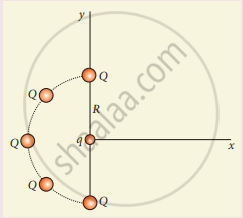Evaluation | Q 4. | Page 75

Suppose a charge +q on Earth’s surface and another +q charge is placed on the surface of the Moon,

1. Calculate the value of q required to balance the gravitational attraction between Earth and Moon
2. Suppose the distance between the Moon and Earth is halved, would the charge q change?
(Take mE = 5.9 x 1024 kg, mM = 7.348 x 1022 kg)
Evaluation | Q 5. (a) | Page 74

Draw the free body diagram for the following charges as shown in the following figure.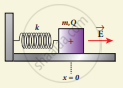Evaluation | Q 5. (b) | Page 74

Draw the free body diagram for the following charges as shown in the following figure.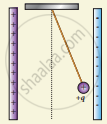Evaluation | Q 5. (c) | Page 74

Draw the free body diagram for the following charges as shown in the following figure.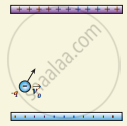Evaluation | Q 6. | Page 75

Consider an electron travelling with a speed VΦ and entering into a uniform electric field vec"E" which is perpendicular to vec"V"_0 as shown in the Figure. Ignoring gravity, obtain the electron’s acceleration, velocity and position as functions of time.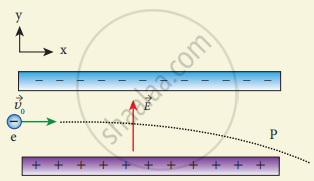Evaluation | Q 7. | Page 76

A closed triangular box is kept in an electric field of magnitude E = 2 × 103 N C-1 as shown in the figure.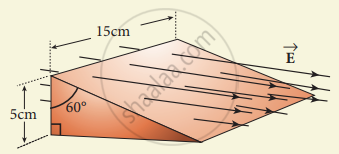Calculate the electric flux through the

1. vertical rectangular surface
2. slanted surface and
3. entire surface.
Evaluation | Q 8. | Page 76

The electrostatic potential is given as a function of x in figure (a) and (b). Calculate the corresponding electric fields in regions A, B, C and D. Plot the electric field as a function of x for figure (b).

1.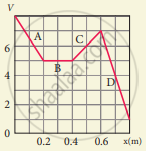2.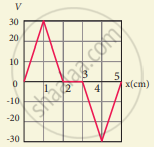Evaluation | Q 9. | Page 76

A spark plug in a bike or a car is used to ignite the air-fuel mixture in the engine. It consists of two electrodes separated by a gap of around 0.6 mm gap as shown in the figure.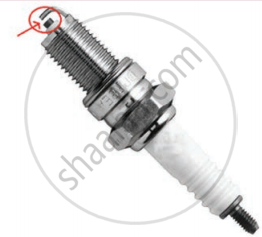To create the spark, an electric field of magnitude 3 x 106Vm-1 is required,

1. What potential difference must be applied to produce the spark?
2. If the gap is increased, does the potential difference increase, decrease or remains the same?
3. Find the potential difference if the gap is 1 mm.
Evaluation | Q 10. | Page 76

A point charge of +10 µC is placed at a distance of 20 cm from another identical point charge of +10 µC. A point charge of -2 µC is moved from point a to b as shown in the figure. Calculate the change in potential energy of the system? Interpret your result.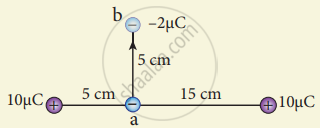Evaluation | Q 11. | Page 77

Calculate the resultant capacitances for each of the following combinations of capacitors.

1.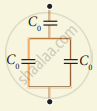2.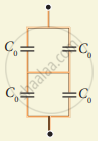3.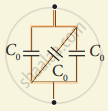4.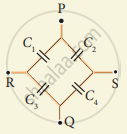5.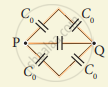Evaluation | Q 12. | Page 77

An electron and a proton are allowed to fall through the separation between the plates of a parallel plate capacitor of voltage 5 V and separation distance h = 1 mm as shown in the figure.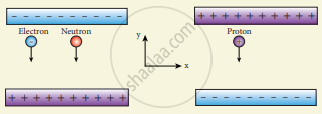1. Calculate the time of flight for both electron and proton.
2. Suppose if a neutron is allowed to fall, what is the time of flight?
3. Among the three, which one will reach the bottom first?

(Take mp = 1.6 x 10-27 kg, me= 9.1 x 10-31 kg and g = 10 m s-2)

Evaluation | Q 13. | Page 78

During a thunder storm, the movement of water molecules within the clouds creates friction, partially causing the bottom part of the clouds to become negatively charged. This implies that the bottom of the cloud and the ground act as a parallel plate capacitor. If the electric field between the cloud and ground exceeds the dielectric breakdown of the air (3 × 106 Vm–1), lightning will occur.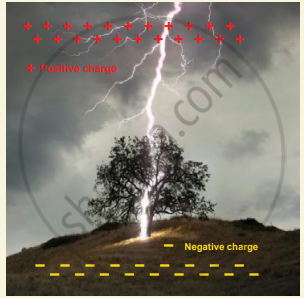1. If the bottom part of the cloud is 1000 m above the ground, determine the electric potential difference that exists between the cloud and ground.
2. In a typical lightning phenomenon, around 25 C of electrons are transferred from cloud to ground. How much electrostatic potential energy is transferred to the ground?
Evaluation | Q 14. | Page 78

For the given capacitor configuration

1. Find the charges on each capacitor
2. potential difference across them
3. energy stored in each capacitor.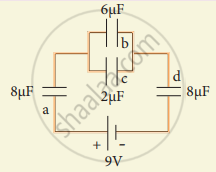Evaluation | Q 15. | Page 78

Capacitors P and Q have identical cross-sectional areas A and separation d. The space between the capacitors is filled with a dielectric of dielectric constant Er as shown in the figure. Calculate the capacitance of capacitors P and Q.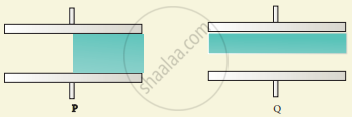## Solutions for Chapter 1: Electrostatics

Evaluation## Tamil Nadu Board Samacheer Kalvi solutions for Class 12th Physics Volume 1 and 2 Answers Guide chapter 1 - Electrostatics

Shaalaa.com has the Tamil Nadu Board of Secondary Education Mathematics Class 12th Physics Volume 1 and 2 Answers Guide Tamil Nadu Board of Secondary Education solutions in a manner that help students grasp basic concepts better and faster. The detailed, step-by-step solutions will help you understand the concepts better and clarify any confusion. Tamil Nadu Board Samacheer Kalvi solutions for Mathematics Class 12th Physics Volume 1 and 2 Answers Guide Tamil Nadu Board of Secondary Education 1 (Electrostatics) include all questions with answers and detailed explanations. This will clear students' doubts about questions and improve their application skills while preparing for board exams.

Further, we at Shaalaa.com provide such solutions so students can prepare for written exams. Tamil Nadu Board Samacheer Kalvi textbook solutions can be a core help for self-study and provide excellent self-help guidance for students.

Concepts covered in Class 12th Physics Volume 1 and 2 Answers Guide chapter 1 Electrostatics are Historical Background of Electric Charges, Basic Properties of Charges, Coulomb’s Law - Force Between Two Point Charges, Electric Field and Electric Field Lines, Electric Dipole and Its Properties, Electrostatic Potential and Potential Energy, Gauss Law and Its Application, Capacitors and Capacitance, Distribution of Charges in a Conductor and Action at Points, Electrostatics of Conductors and Dielectrics.

Using Tamil Nadu Board Samacheer Kalvi Class 12th Physics Volume 1 and 2 Answers Guide solutions Electrostatics exercise by students is an easy way to prepare for the exams, as they involve solutions arranged chapter-wise and also page-wise. The questions involved in Tamil Nadu Board Samacheer Kalvi Solutions are essential questions that can be asked in the final exam. Maximum Tamil Nadu Board of Secondary Education Class 12th Physics Volume 1 and 2 Answers Guide students prefer Tamil Nadu Board Samacheer Kalvi Textbook Solutions to score more in exams.

Get the free view of Chapter 1, Electrostatics Class 12th Physics Volume 1 and 2 Answers Guide additional questions for Mathematics Class 12th Physics Volume 1 and 2 Answers Guide Tamil Nadu Board of Secondary Education, and you can use Shaalaa.com to keep it handy for your exam preparation.